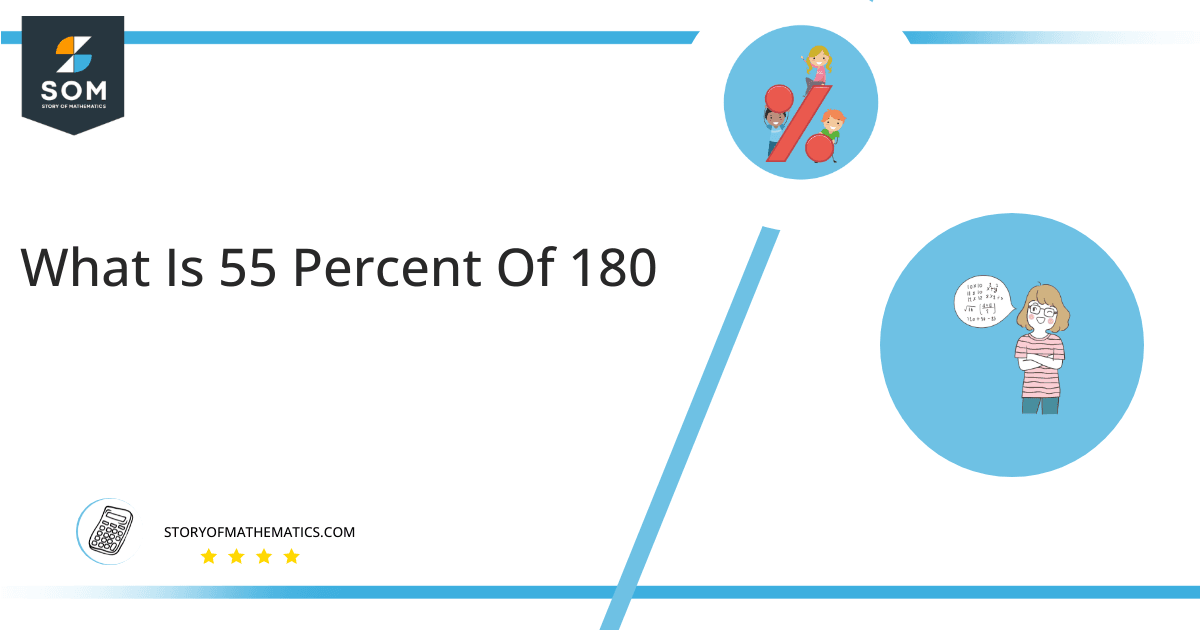# What Is 55 Percent of 180 + Solution with Free Steps?

The 55 percent of 180 is equal to 99. It can be easily calculated by dividing 55 by 100 and multiplying the answer with 180 to get 99.The easiest way to get this answer is by solving a simple mathematical problem of percentages. You need to find 55% of 180 for some sale or real-life problem. Divide 55 by 100, multiply the answer by 180, and get the 55% of 180 value in seconds.

This article will explain the full process of finding any percentage value from any given quantity or number with easy and simple steps.

## What Is 55 percent of 180?

The 55 percent of 180 is 99.

The percentage can be understood with a simple explanation. Take 180, and divide it into 100 equal parts. The 55 parts from the total of 100 parts is called 55 percent, which is 99 in this example.

## How To Calculate 55 percent of 180?

You can find 55 percent of 180 by some simple mathematical steps explained below.### Step 1

Firstly, depict 55 percent of 180 as a fractional multiple as shown below:

55% x 180

### Step 2

The percentage sign % means percent, equivalent to the fraction of 1/100.

Substituting this value in the above formula:

= (55/100) x 180

### Step 3

Using the algebraic simplification process, we can arithmetically manipulate the above equation as follows:

= (55 x 180) / 100

= 9900 / 100

= 99This percentage can be represented on a pie chart for visualization. Let us suppose that the whole pie chart represents the 180 values. Now, we find 55 percent of 180, which is 99. The area occupied by the 99 value will represent the 55 percent of the total 180 values. The remaining region of the pie chart will represent 45 percent of the total 180 values. The 100% of 180 will cover the whole pie chart as 180 is the total value.

Any given number or quantity can be represented in percentages to understand the total quantity better. The percentage can be considered a quantity that divides any number into hundred equal parts for better representation of large numbers and understanding.

Percentage scaling or normalization is a very simple and convenient method of representing numbers in relative terms. Such notations find wide application in many industrial sectors where the relative proportions are used.